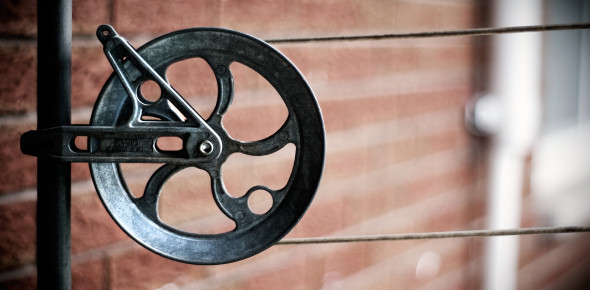# Simple Machines Quiz: Test Your Knowledge!

28 Questions | Attempts: 647
ShareSettings.

• 1.
If you bite into an apple, your teeth would be considered what type of simple machine?
• A.

Inclined plane

• B.

Wheel and axle

• C.

Wedge

• D.

Pulley

• 2.
What is the main purpose of a wedge? (work it does)
• A.

Push things apart

• B.

Push things together

• C.

Move away from objects

• D.

Lift objects

• 3.
The sharp edge of a knife is considered to be a wedge.
• A.

True

• B.

False

• 4.
This is necessary to get an object moving...
• A.

Friction

• B.

Force

• C.

Resistance

• D.

Wedge

• 5.
A rod attached to the center of a wheel is called:
• A.

Magnet

• B.

Wedge

• C.

Inclined plane

• D.

Axle

• 6.
The efficiency of a simple machine will always be 100% or higher.
• A.

True

• B.

False

• 7.
Cranes, flagpoles, and window blinds are examples of what type of simple machines?
• A.

Inclined Planes

• B.

Wedge

• C.

Pulley

• D.

Wheel and axle

• 8.
What Ancient civilization likely used the inclined plane to build the pyramids?
• A.

Egyptians

• B.

British

• C.

French

• D.

Greeks

• 9.
When you increase the distance of a moving object on an inclined plane ...
• A.

Less force is needed to move an object

• B.

More force is needed to move an object

• C.

The amount of force does not matter

• D.

There is no reason to worry about the longer distance, because it will not make a difference in the amount of work is done

• 10.
Using multiple pulleys would reduce the amount of force needed in order to do the work of the object.
• A.

True

• B.

False

• 11.
What type of simple machine is found on the cap of a pickle jar?
• A.

Wedge

• B.

Wheel

• C.

Screw

• D.

Pulley

• 12.
A device that has two or more simple machines working together
• A.

Complete machine

• B.

Independent machine

• C.

Complex machine

• D.

Simple machine

• 13.
Work  is done when a force is applied over a distance.
• A.

True

• B.

False

• 14.
Screws and wedges are examples of an inclined plane.
• A.

True

• B.

False

• 15.
Which simple machine would most likely be used to move an object over great distances and on a flat surface?
• A.

Wedge

• B.

Wheel and axle

• C.

Inclined plane

• D.

Pulley

• 16.
A door knob would be what type of simple machine?
• A.

Wheel and axle

• B.

Pulley

• C.

Lever

• D.

Wedge

• 17.
Which of the following items would be considered as a(n) wheel and axle?
• A.

Nutcracker

• B.

Axe

• C.

Windmill

• D.

Screwdriver

• 18.
The spokes on the wheel of a bicycle are actually like the arms of a lever.
• A.

True

• B.

False

• 19.
What simple machine would be designed in order to take a fish out of the water easily with a fishing pole?
• A.

Lever

• B.

Wedge

• C.

Inclined plane

• D.

Pulley

• 20.
Which of the following would not be using mechanical advantage
• A.

Using your hand to open a jar

• B.

Using a pulley to raise a flag on a flagpole

• C.

Use a crowbar to lift a heavy rock

• D.

Using an axe to cut through a log

• 21.
The simple machine of a lever is used to move an elevator.
• A.

True

• B.

False

• 22.
Which of the following would not be an example of a screw, simple machine object?
• A.

Nuts and bolts

• B.

Jar lid

• C.

Light bulb

• D.

Wheelbarrow

• 23.
When a screwdriver is used to open a can of paint, what is the fulcrum in this specific example?
• A.

• B.

The edge of the paint can

• C.

Lid of the paint can

• D.

The screwdriver

• 24.
Where would you find the fulcrum on a seesaw?
• A.

On the left side

• B.

On the right side

• C.

In the middle

• D.

On the top

• 25.
The fulcrum of a lever is the same as an axle on the wheel and axle, simple machine.
• A.

True

• B.

False

## Related TopicsBack to top
×

Wait!
Here's an interesting quiz for you.